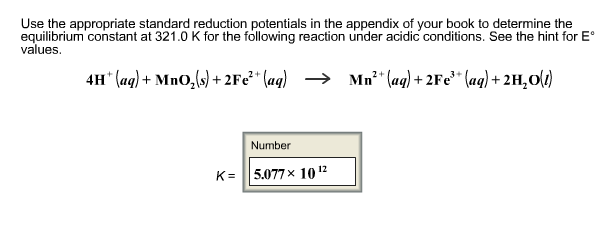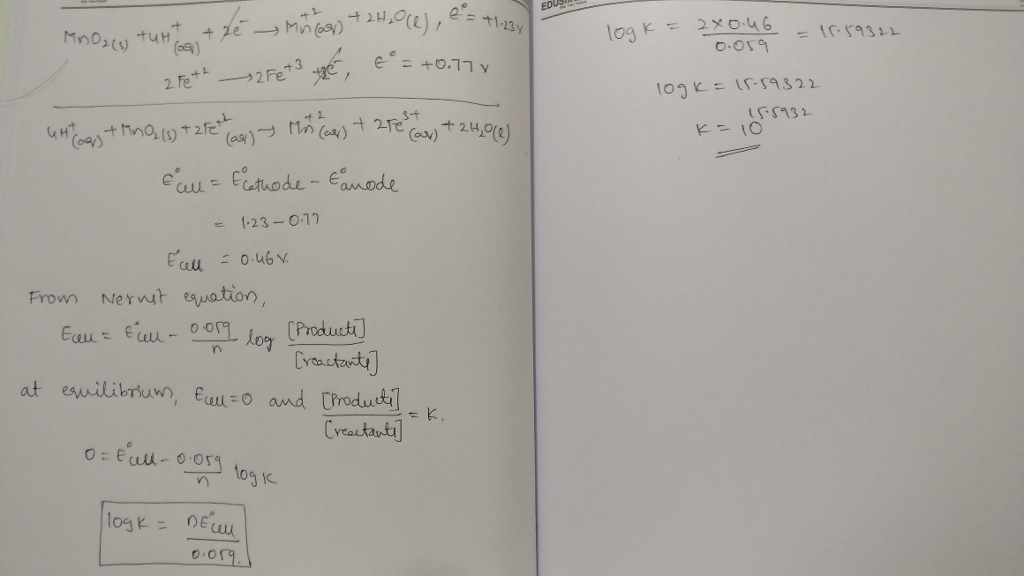# Question & Answer: Use the appropriate standard reduction potentials in the appendix of your book to…..Use the appropriate standard reduction potentials in the appendix of your book to determine the equilibrium constant at 321.0 K for the following reaction under acidic conditions. See the hint for E^compositefunction values 4H^+(aq) + MnO_2(s) + 2Fe^2+ + (aq) rightarrow Mn^2+ (aq) + 2Fe^3+ (aq) + 2H_2O(l) K = 5.077 times 10^12